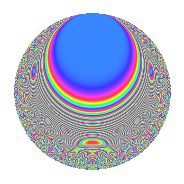# Properties

 Label 1001.2.aLevel 1001 Weight 2 Character orbit a Rep. character $$\chi_{1001}(1,\cdot)$$ Character field $$\Q$$ Dimension 59 Newform subspaces 14 Sturm bound 224 Trace bound 3

# Related objects

## Defining parameters

 Level: $$N$$ = $$1001 = 7 \cdot 11 \cdot 13$$ Weight: $$k$$ = $$2$$ Character orbit: $$[\chi]$$ = 1001.a (trivial) Character field: $$\Q$$ Newform subspaces: $$14$$ Sturm bound: $$224$$ Trace bound: $$3$$ Distinguishing $$T_p$$: $$2$$, $$3$$

## Dimensions

The following table gives the dimensions of various subspaces of $$M_{2}(\Gamma_0(1001))$$.

Total New Old
Modular forms 116 59 57
Cusp forms 109 59 50
Eisenstein series 7 0 7

The following table gives the dimensions of the cuspidal new subspaces with specified eigenvalues for the Atkin-Lehner operators and the Fricke involution.

$$7$$$$11$$$$13$$FrickeDim.
$$+$$$$+$$$$+$$$$+$$$$5$$
$$+$$$$+$$$$-$$$$-$$$$8$$
$$+$$$$-$$$$+$$$$-$$$$12$$
$$+$$$$-$$$$-$$$$+$$$$5$$
$$-$$$$+$$$$+$$$$-$$$$8$$
$$-$$$$+$$$$-$$$$+$$$$5$$
$$-$$$$-$$$$+$$$$+$$$$5$$
$$-$$$$-$$$$-$$$$-$$$$11$$
Plus space$$+$$$$20$$
Minus space$$-$$$$39$$

## Trace form

 $$59q - 3q^{2} + 69q^{4} - 2q^{5} + 4q^{6} - q^{7} - 15q^{8} + 51q^{9} + O(q^{10})$$ $$59q - 3q^{2} + 69q^{4} - 2q^{5} + 4q^{6} - q^{7} - 15q^{8} + 51q^{9} - 2q^{10} + 7q^{11} - 12q^{12} - q^{13} - 3q^{14} + 12q^{15} + 77q^{16} - 10q^{17} + 17q^{18} + 20q^{19} + 30q^{20} - 4q^{21} - 3q^{22} - 8q^{23} + 44q^{24} + 45q^{25} - 3q^{26} - 12q^{27} - 7q^{28} - 18q^{29} + 24q^{30} + 12q^{31} - 23q^{32} + 26q^{34} - 2q^{35} + 89q^{36} - 10q^{37} + 12q^{38} - 4q^{39} + 6q^{40} - 18q^{41} + 28q^{42} - 32q^{43} + 13q^{44} - 22q^{45} + 24q^{46} - 16q^{47} - 28q^{48} + 59q^{49} - 53q^{50} - 24q^{51} - 7q^{52} + 30q^{53} - 56q^{54} + 6q^{55} - 15q^{56} - 64q^{57} - 82q^{58} - 32q^{59} + 32q^{60} + 10q^{61} - 40q^{62} + 3q^{63} + 117q^{64} - 2q^{65} - 12q^{66} - 8q^{67} - 70q^{68} + 44q^{69} - 10q^{70} + 76q^{71} - 43q^{72} - 2q^{73} - 2q^{74} + 60q^{75} + 36q^{76} - q^{77} - 4q^{78} + 36q^{79} + 6q^{80} + 19q^{81} - 46q^{82} + 28q^{83} - 28q^{84} + 12q^{85} + 20q^{86} + 136q^{87} - 15q^{88} - 38q^{89} - 34q^{90} + 7q^{91} - 40q^{92} + 12q^{93} + 32q^{94} + 44q^{95} + 52q^{96} - 6q^{97} - 3q^{98} + 31q^{99} + O(q^{100})$$

## Decomposition of $$S_{2}^{\mathrm{new}}(\Gamma_0(1001))$$ into newform subspaces

Label Dim. $$A$$ Field CM Traces A-L signs $q$-expansion
$$a_2$$ $$a_3$$ $$a_5$$ $$a_7$$ 7 11 13
1001.2.a.a $$1$$ $$7.993$$ $$\Q$$ None $$-2$$ $$-3$$ $$-3$$ $$-1$$ $$+$$ $$-$$ $$+$$ $$q-2q^{2}-3q^{3}+2q^{4}-3q^{5}+6q^{6}+\cdots$$
1001.2.a.b $$1$$ $$7.993$$ $$\Q$$ None $$-1$$ $$0$$ $$-2$$ $$-1$$ $$+$$ $$-$$ $$+$$ $$q-q^{2}-q^{4}-2q^{5}-q^{7}+3q^{8}-3q^{9}+\cdots$$
1001.2.a.c $$1$$ $$7.993$$ $$\Q$$ None $$0$$ $$2$$ $$-1$$ $$-1$$ $$+$$ $$+$$ $$+$$ $$q+2q^{3}-2q^{4}-q^{5}-q^{7}+q^{9}-q^{11}+\cdots$$
1001.2.a.d $$2$$ $$7.993$$ $$\Q(\sqrt{5})$$ None $$0$$ $$3$$ $$3$$ $$2$$ $$-$$ $$+$$ $$+$$ $$q+(1-2\beta )q^{2}+(2-\beta )q^{3}+3q^{4}+(1+\cdots)q^{5}+\cdots$$
1001.2.a.e $$2$$ $$7.993$$ $$\Q(\sqrt{21})$$ None $$2$$ $$1$$ $$-1$$ $$2$$ $$-$$ $$+$$ $$+$$ $$q+q^{2}+(1-\beta )q^{3}-q^{4}-\beta q^{5}+(1-\beta )q^{6}+\cdots$$
1001.2.a.f $$3$$ $$7.993$$ 3.3.1101.1 None $$3$$ $$-1$$ $$1$$ $$-3$$ $$+$$ $$-$$ $$+$$ $$q+q^{2}-\beta _{1}q^{3}-q^{4}+(1-\beta _{1}+\beta _{2})q^{5}+\cdots$$
1001.2.a.g $$4$$ $$7.993$$ 4.4.23301.1 None $$-2$$ $$-1$$ $$-5$$ $$4$$ $$-$$ $$+$$ $$+$$ $$q+(-1+\beta _{3})q^{2}-\beta _{1}q^{3}+(2-\beta _{2})q^{4}+\cdots$$
1001.2.a.h $$4$$ $$7.993$$ 4.4.1957.1 None $$0$$ $$-1$$ $$5$$ $$-4$$ $$+$$ $$+$$ $$+$$ $$q+(-\beta _{1}-\beta _{2}-\beta _{3})q^{2}+(\beta _{1}+\beta _{2})q^{3}+\cdots$$
1001.2.a.i $$5$$ $$7.993$$ 5.5.106069.1 None $$-2$$ $$-3$$ $$0$$ $$5$$ $$-$$ $$+$$ $$-$$ $$q-\beta _{1}q^{2}+(-1-\beta _{3})q^{3}+(\beta _{1}+\beta _{2}+\cdots)q^{4}+\cdots$$
1001.2.a.j $$5$$ $$7.993$$ 5.5.216637.1 None $$-2$$ $$0$$ $$-4$$ $$-5$$ $$+$$ $$-$$ $$-$$ $$q-\beta _{1}q^{2}-\beta _{3}q^{3}+(\beta _{1}+\beta _{2})q^{4}+(-1+\cdots)q^{5}+\cdots$$
1001.2.a.k $$5$$ $$7.993$$ 5.5.81509.1 None $$0$$ $$-4$$ $$-6$$ $$5$$ $$-$$ $$-$$ $$+$$ $$q+\beta _{4}q^{2}+(-1+\beta _{2})q^{3}+(1-\beta _{2}+\beta _{3}+\cdots)q^{4}+\cdots$$
1001.2.a.l $$7$$ $$7.993$$ $$\mathbb{Q}[x]/(x^{7} - \cdots)$$ None $$0$$ $$6$$ $$9$$ $$-7$$ $$+$$ $$-$$ $$+$$ $$q+\beta _{5}q^{2}+(1-\beta _{1})q^{3}+(4+\beta _{1}-\beta _{2}+\cdots)q^{4}+\cdots$$
1001.2.a.m $$8$$ $$7.993$$ 8.8.3212905625.1 None $$2$$ $$-1$$ $$-5$$ $$-8$$ $$+$$ $$+$$ $$-$$ $$q+\beta _{7}q^{2}-\beta _{2}q^{3}+(2-\beta _{3})q^{4}+(-1+\cdots)q^{5}+\cdots$$
1001.2.a.n $$11$$ $$7.993$$ $$\mathbb{Q}[x]/(x^{11} - \cdots)$$ None $$-1$$ $$2$$ $$7$$ $$11$$ $$-$$ $$-$$ $$-$$ $$q-\beta _{1}q^{2}-\beta _{8}q^{3}+(1+\beta _{2})q^{4}+(1-\beta _{4}+\cdots)q^{5}+\cdots$$

## Decomposition of $$S_{2}^{\mathrm{old}}(\Gamma_0(1001))$$ into lower level spaces

$$S_{2}^{\mathrm{old}}(\Gamma_0(1001)) \cong$$ $$S_{2}^{\mathrm{new}}(\Gamma_0(11))$$$$^{\oplus 4}$$$$\oplus$$$$S_{2}^{\mathrm{new}}(\Gamma_0(77))$$$$^{\oplus 2}$$$$\oplus$$$$S_{2}^{\mathrm{new}}(\Gamma_0(91))$$$$^{\oplus 2}$$$$\oplus$$$$S_{2}^{\mathrm{new}}(\Gamma_0(143))$$$$^{\oplus 2}$$